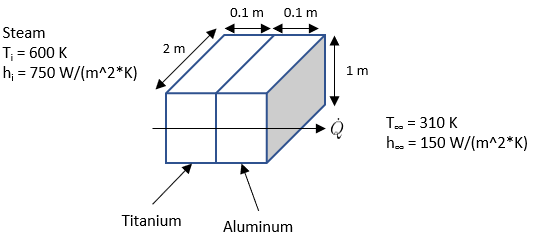## Thermal Resistance

In the figure below, two metals plates are stacked side-by-side, with hot steam on one end, and ambient air on the other. If Titanium has a thermal conductivity of k = 50 W/m∙K, and Aluminum has k = 240 W/m∙K, what is the heat flux through the plate system?Hint
Thermal Resistance:
$$\dot{Q}=\frac{\Delta T}{R_{total}}$$$where $$\dot Q$$ is the rate of heat transfer in Watts, $$\Delta T$$ is the change in temperature in Kelvin, and $$R_{total}$$ is the resistances in series. Hint 2 Plane Wall Conduction Resistance is $$R=\frac{L}{kA}$$$
where $$L$$ is the wall thickness, $$k$$ is the thermal conductivity, and $$A$$ is the convection surface area. For convection resistance:
$$R=\frac{1}{hA}$$$where $$h$$ is the convection heat-transfer coefficient of the fluid, and $$A$$ is the convection surface area. Because there are two different material plates adjacent to each other, we have thermal resistances in series: $$\dot{Q}=\frac{\Delta T}{R_{total}}$$$
where $$\dot Q$$ is the rate of heat transfer in Watts, $$\Delta T$$ is the change in temperature in Kelvin, and $$R_{total}$$ is the resistances in series. Conduction is occurring within the contacting plates, and convection is occurring between the steam/Titanium and the Aluminum/ambient environment. Resistances in series are added: $$R_{total}=\Sigma R$$ , where Plane Wall Conduction Resistance is
$$R=\frac{L}{kA}$$$where $$L$$ is the wall thickness, $$k$$ is the thermal conductivity, and $$A$$ is the convection surface area. For convection resistance: $$R=\frac{1}{hA}$$$
where $$h$$ is the convection heat-transfer coefficient of the fluid, and $$A$$ is the convection surface area. Thus,
$$R_{total}=\frac{1}{A}[\frac{1}{h_i}+\frac{L_{Ti}}{k_{Ti}}+\frac{L_{Al}}{k_{Al}}+\frac{1}{h_\infty}]$$$$$=\frac{1}{(2m)(1m)}[\frac{1}{750W/m^2\cdot K}+\frac{0.1m}{50W/m\cdot K}+\frac{0.1m}{240W/m\cdot K}+\frac{1}{150W/m^2\cdot K}]$$$
$$=\frac{1}{2m^2}[0.00133\frac{m^2\cdot K}{W}+0.002\frac{m^2\cdot K}{W}+0.000416\frac{m^2\cdot K}{W}+0.00667\frac{m^2\cdot K}{W}]$$$$$R_{total}=\frac{1}{2}[0.0104\frac{K}{W}]=0.0052\:\frac{K}{W}$$$
To find the heat rate:
$$\dot{Q}=\frac{(T_{i}-T_{\infty })}{0.0052\frac{K}{W}}=\frac{(600K-310K)W}{0.0052K}=55,769\:W$$$Since heat flux is $$q_x"=\dot Q/Area$$ $$q_x"=\frac{55,769W}{(2m)(1m)}=27,885\:\frac{W}{m^2}$$$
$$27,885\:W/m^2$$\$